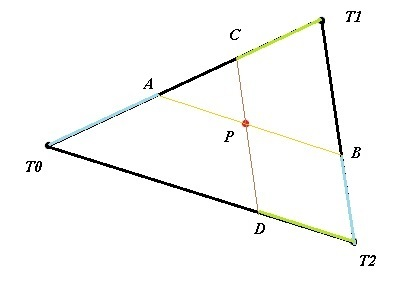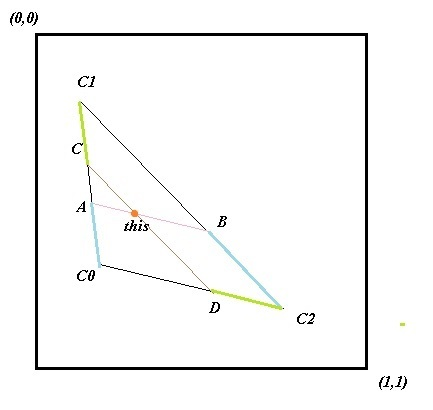# Raycast to texture coord (or: 3d point to texture coord)?

Hi all,

Anyone got a tutorial or knows how to convert a 3d position to the 2d texture coord on a quad (assume the vector is on quad).

Basically what I want to do is this:

See the cursor pointing on the wall on the left? I know how to do ray casting from camera and cursor and get the exact 3d point on the wall I point on, but how do I then convert that position to the 2d position on the texture on the right?

To make things easy - its all just simple quads with UV of 0, 0, 1, 1, no special mapping or atlas.

EDIT: this explains best what I want to do: https://docs.unity3d.com/ScriptReference/RaycastHit-textureCoord.html

Thanks!

How are your quads represented?

I’ve previously written a ray tracer and I found it’s easiest to have primitives be as simple as possible and allow for a transformation matrix to do the hard work. When ray tracing I transformed the ray into local space and resolved the collision check against the simple version of the primitive. For example, for a quad the primitive would be a square defined by two vectors of unit length along the x and y axes. If you transform rays into that local space, the collision coordinates will already be the UV coordinates.

If you represent your quads in another way, you need to do a change of basis from world space to the unit square space mentioned above. To do that, first create matrix with as first two columns the vectors along the sides of your quad (horizontal and vertical respectively), as third column the cross product of the first two vectors and as fourth column the bottom left (in local space, so the origin of the vectors) of the quad. These are all 3d vectors, so the last row isn’t filled yet. That should be 0 0 0 1 (for anyone unfamiliar with the underlying maths, this is needed for the translation; check out Wikipedia on transformation matrices and homogeneous coordinates if you’re interested). That’s the transformation matrix from local space to world space. If you invert it and transform your point with the result, the x and y coordinates of the transformed vector will be the UVs.

There’s probably some simplifications you can make to this calculation because you don’t need the z coordinate of the result and if you track the inverse transform when building the quad you never need to explicitly compute the inverse transform. If you write out the whole computation it’s easy to figure out the simplified version. Though it’s surely somewhere on the internet alreadyTracking inverse transform for basic transformations is pretty easy. For rotation rotate the same amount but the other direction, for translation translate with negated sign and for scaling scale by the inverse. You can use the `Matrix.CreateX` methods for those.

Sorry if this was a bit incoherent, I’m on mobileHope that helps!1 Like

To add.

If you are already triangle picking and calculate from ‘areal coordinate’ x,y,z positions the corresponding u,v texel coordinates for a point on that triangle 0 to 1, 0 to 1 (which is the hard part).
Then the corresponding (image / texture) indexing cartesian coordinates are simply.
texturePosition.X = u * texture.Width;
texturePosition.Y = v * texture.Height;

I don’t remember if this one gives the point but i seem to remember thinking it would be easy enough to do it.
http://xbox.create.msdn.com/en-us/education/catalog/sample/picking_triangle

Do look at this one there are non parametric solutions as well im pretty sure.
http://geomalgorithms.com/a06-_intersect-2.html

google “ray triangle intersection”

1 Like

Thanks @Jjagg and @willmotil,
Sorry for the late response I haven’t had much time to work on it but your answers look helpful, hopefully I’ll have the time to test them tomorrow.for ex:
vertex0:(position: T0, texcoord:C0)
vertex1:(position: T1, texcoord:C1)
vertex2:(position: T2, texcoord:C2)

Triangle:P: collision point
r1 = AT0/T1T0 = BT2/T1T2
r2 = CT1/T1T0 = DT2/T0T2

Texture:AC0 = C1C0r1
BC2 = C1C2
r1

CC1 = C1C0r2
DC2 = C0C2
r2

result is the intersection of AB and CD

anyone got it work?

I never tried the above, but one time I needed the UV coord when using an atlas(auto-generated) for a 3D paint prog I made. I made a render-target and used vertex colors so the R channel would tell me the U coordinate and the G channel would tell be the V coordinate of whatever was under the mouse (and then used sphere falloff with visibility testing to air-brush out from it). I think the only downside was you needed to lock the target to find the color and thereby get the UV coord on the CPU side [tiny performance hit]. You’d only want to check one pixel, so in Monogame you’d probably want to use the appropriate GetData overload and allocate for 1 pixel ie: pixCol = new Color - choose start address and span of 1.
Not sure if this is what anyone would want to do - but just putting it out there in case.